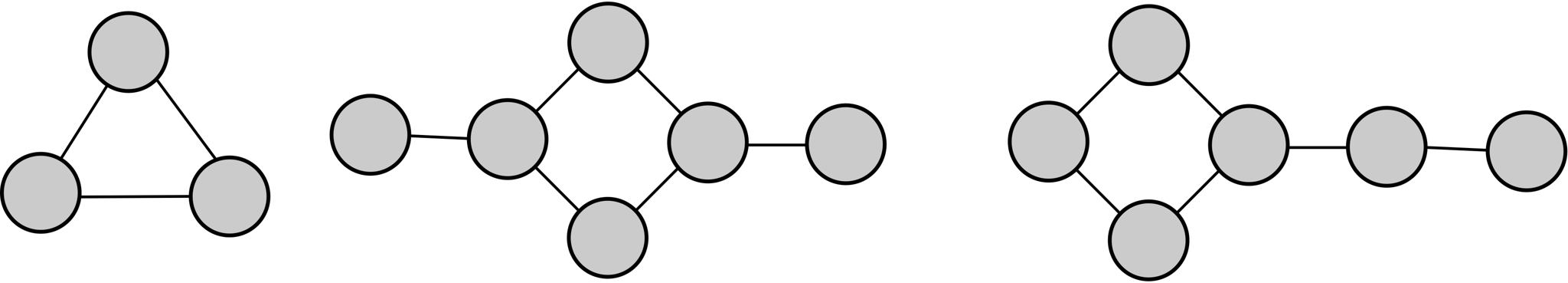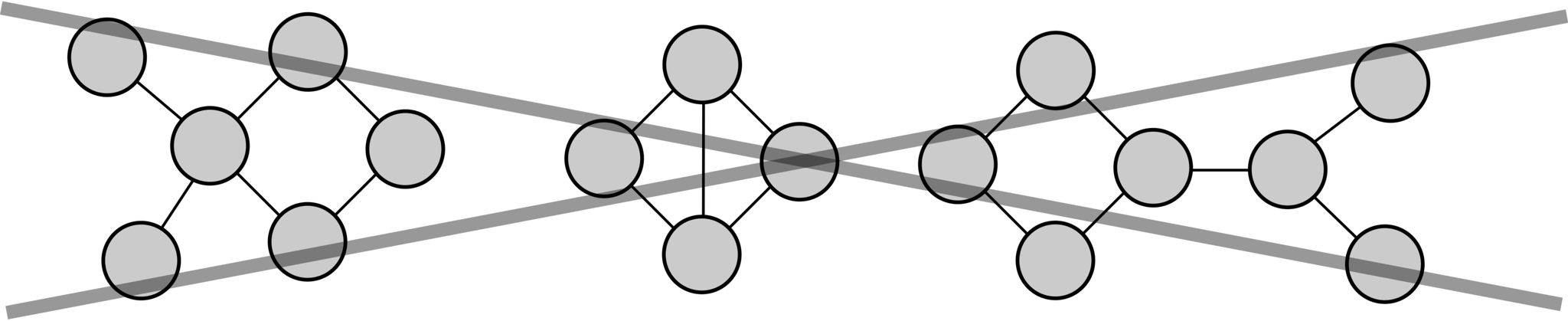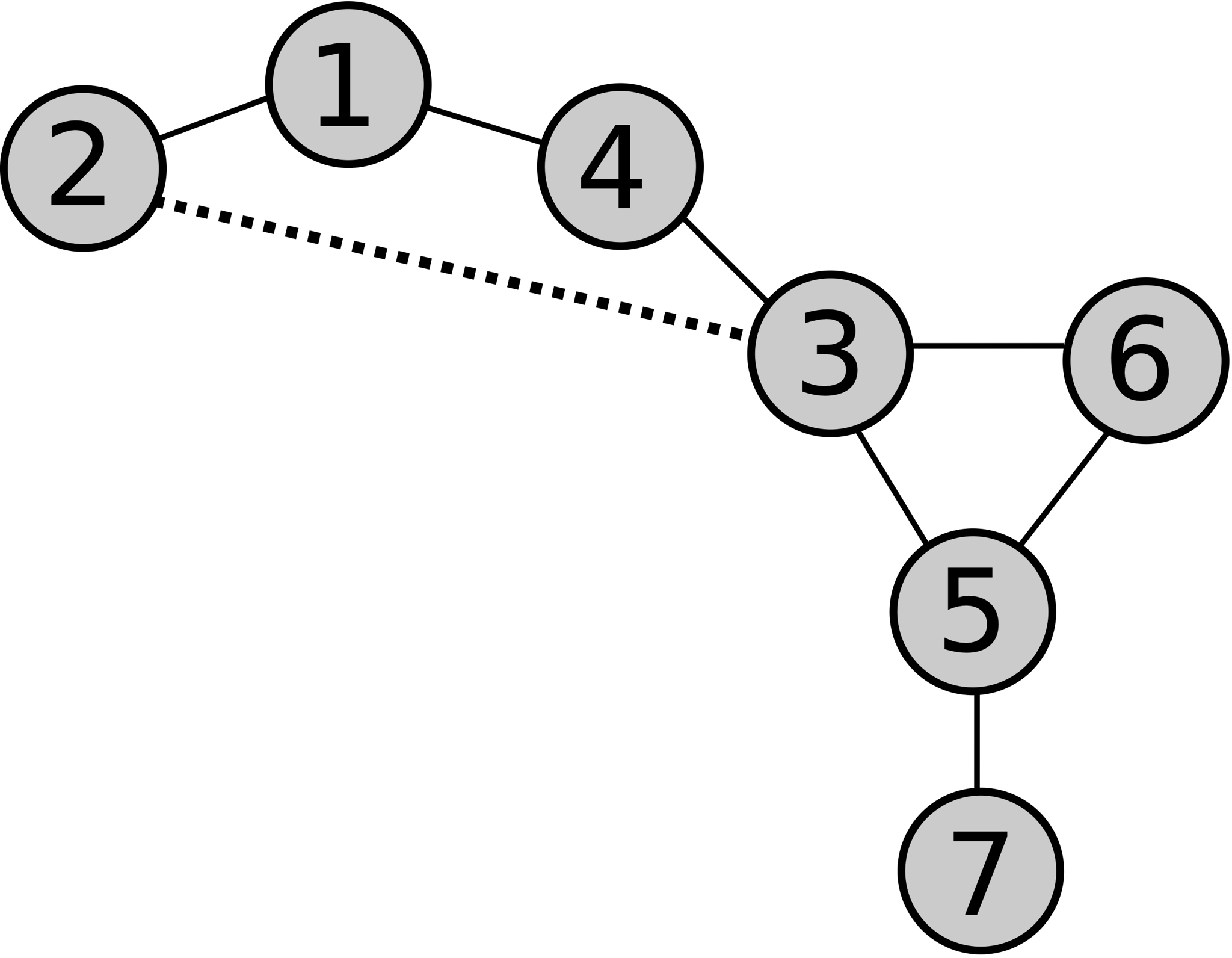시간 제한 메모리 제한 제출 정답 맞은 사람 정답 비율
2 초 256 MB 2 2 2 100.000%

## 문제

Vlad is engaged in underwater photography. Most of all he likes photographing squids and octopuses. For example, did you know that an octopus has three hearts? And the fact that they can change the shape and color of the body? And here is one more astonishing... Oh, come on, Vlad, get away from the keyboard, and enough to write about the octopuses!

Vlad is not only a photographer, but also an excellent programmer. He developed a special program for automatic octopus recognition in photographs. The program receives a photograph as the input. And as the output, it gives a representation of octopus in the form of an undirected graph. Informally, an undirected graph is a set of points and a set of edges connecting them. The graph obtained by recognizing an octopus image always has a specific form: three or more points form the body, and the remaining points are parts of tentacles which are attached to body points so that no more than one tentacle is attached to each point of the body. The body is a sequence of points in which any two adjacent points, as well as the first and the last points, are connected by an edge, and the number of edges connected to each point is either two or three (if a tentacle is attached to it). A tentacle is a sequence of points in which any two adjacent points are connected by an edge, and the number of edges connected to each point is either two or one (in each tentacle, there is exactly one point with only one edge). Additionally, in this graph, there is no more than one edge between any two points, and every edge connects two different points.

When Vlad collected all his photographs, he launched the recognition process. After several hours of waiting, he got the result. However, because of a tricky bug in the code, one extra edge was added to all the graphs! After rereading the source code of his program, Vlad realized that exactly one edge was indeed added to each graph. Moreover, the added edge connects two different points which were not previously directly connected by an edge. Help Vlad to find the extra edge, and in return, he will tell you more amazing facts about octopuses!

## 입력

The first line contains integers $n$ and $m$ separated by a space: the number of points and the number of edges in the graph ($1 \le n, m \le 10^5$).

The following $m$ lines describe the edges of the graph. Each edge is given by two different integers separated by a space: the numbers of points connected by this edge. The points are numbered starting from one. Each pair of points occurs no more than once.

It is guaranteed that the given graph was obtained by adding exactly one edge to a graph resulting from an octopus image recognition.

## 출력

In a single line, output two integers separated by a space: the numbers of the points between which you need to remove the edge. You can output the numbers in any order.

If there are several correct answers, output any one of them.

## 예제 입력 1

7 8
1 2
2 3
3 4
4 1
3 5
5 6
5 7
3 6


## 예제 출력 1

3 2


## 힌트

Here are examples of graphs which are correct representations of octopuses:Here are examples of graphs which are not correct representations of octopuses:Illustration of the first test: